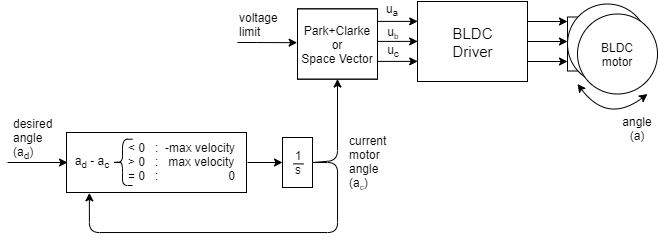# Position open-loop control

This control loop allows you to move your motor to the desired angle in real time without using the position sensor. This mode is enabled by:

``````// set position motion control open-loop
motor.controller = MotionControlType::angle_openloop;
``````You can test this algorithm by running the examples in the `motion_control/open_loop_motor_control/` folder.

This control algorithm is very simple. User sets the target angle it wants to achieve ad. The algorithm only subtracts the current angle ac and the desired angle ad to find the direction it needs to move and goes in that direction with the highest velocity possible `motor.velocity_limit`(max velocity). To set this velocity it uses the same algorithm as for velocity open-loop control. It integrates the velocity it in time to find out what is the angle it needs to set to the motor ac in order to achieve it. Then the maximal allowed voltage `motor.voltage_limit` is going to be applied in the direction of the ac using `SinePWM` or `SpaceVector` modulation.

## Configuration

``````// choose FOC modulation (optional)
motor.foc_modulation = FOCModulationType::SpaceVectorPWM;

//  maximal velocity of the position control
// default 20
motor.velocity_limit = 20;

// limiting voltage
motor.voltage_limit = 3;   // Volts
// or current  - if phase resistance provided
motor.current_limit = 0.5 // Amps
``````

This type of motion control is highly inefficient therefore try not to use to high value for `motor.voltage_limit`. We suggest you to provide the motor class with the `phase_resistance` value and set the `motor.current_limit` instead the voltage limit. This current might be surpassed but at least you will know an approximate current your motor is drawing. You can calculate the current the motor is going to be producing by checking the motor resistance `phase_resistance` and evaluating:

``````voltage_limit = current_limit * phase_resistance; // Amps
``````

The maximal velocity `motor.velocity_limit` value is going to determine how fast your motor goes in between positions. The higher the value the faster the transition. But since we are turning the motor in open-loop we will not be able to know if the motor can follow the velocity. So make sure to put the `velocity_limit` value that is achievable for your motor. Also beware that for higher velocities and more holding torque you will need to increase the `motor.voltage_limit` or `motor.current_limit` variable as well.

Also, you can change the voltage limit `motor.voltage_limit` (`motor.current_limit`) and transition velocity `motor.velocity_limit` in real-time if you need this kind of behavior in your application.

## Position open-loop control example

Here is one basic example of the velocity open-loop control with the complete configuration. The program will set the target position of `0 RAD` and maintain it, and the user can change the target position using serial terminal.

``````// Open loop motor control example
#include <SimpleFOC.h>

// BLDC motor & driver instance
BLDCMotor motor = BLDCMotor(11);
BLDCDriver3PWM driver = BLDCDriver3PWM(9, 5, 6, 8);

//target variable
float target_position = 0;

// instantiate the commander
Commander command = Commander(Serial);
void doTarget(char* cmd) { command.variable(&target_position, cmd); }

void setup() {

// driver config
// power supply voltage [V]
driver.voltage_power_supply = 12;
driver.init();
// link the motor and the driver

// limiting motor movements
motor.voltage_limit = 3;   // [V]
motor.velocity_limit = 5; // [rad/s] cca 50rpm
// open loop control config
motor.controller = MotionControlType::angle_openloop;

// init motor hardware
motor.init();

Serial.begin(115200);